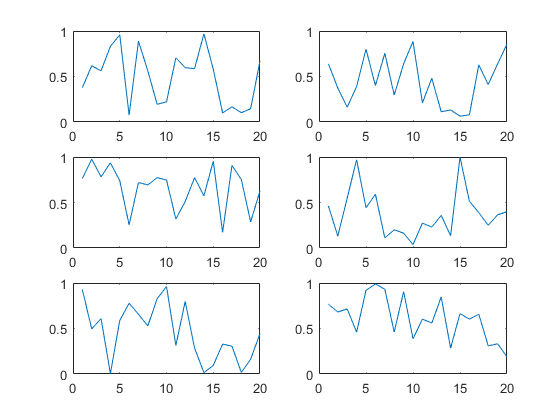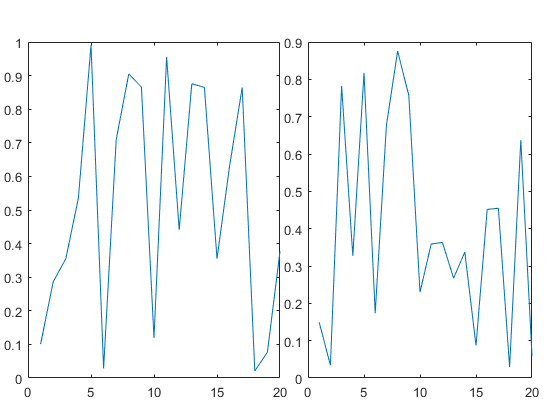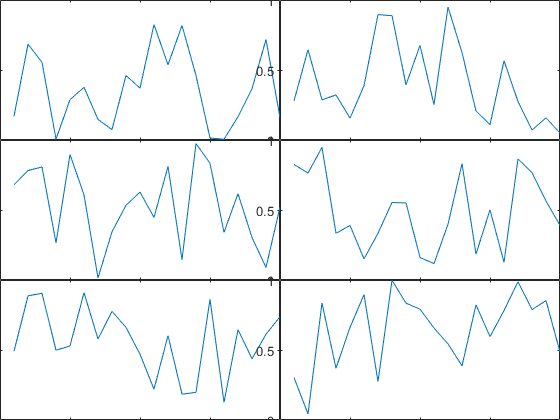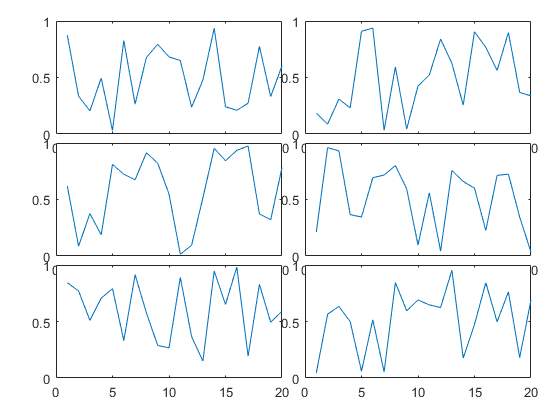# Subplot Position Calculator

Jiro‘s pick this week is Subplot Position Calculator by Christopher Hummersone.

subplot is a nice way to place a grid of axes on a single figure. But the spacing is sometimes a bit too generous, leaving too little room for the graph.

nRows = 3;
nCols = 2;
for m = 1:nRows
for n = 1:nCols
subplot(nRows,nCols,(m-1)*nCols+n)
plot(rand(20,1))
end
endIn that case, you can use axes or subplot to specify the actual placement of the axes.

figure
subplot('position',[0.05 0.1 0.45 0.8])
plot(rand(20,1))
subplot('position',[0.55 0.1 0.4 0.8])
plot(rand(20,1))But this defeats the purpose of subplot because you have to calculate and specify the position manually. This is where Christopher’s entry comes in handy. It lets you specify the grid layout, with optional parameters, and it returns a set of position vectors which you can feed into subplot or axes.

The default is a tight layout with no margin.

pos = iosr.figures.subfigrid(nRows,nCols);
figure
for m = 1:nRows
for n = 1:nCols
subplot('Position',pos(m,:,n))
plot(rand(20,1))
end
endYou can specify the spacing and the scaling by passing in a couple of optional arguments.

pos = iosr.figures.subfigrid(nRows,nCols,[0.1 0.05 0.05 0.1],[.95 .95]);
figure
for m = 1:nRows
for n = 1:nCols
subplot('Position',pos(m,:,n))
plot(rand(20,1))
end
endAs some of you may have guessed, his function is part of a package (IoSR Matlab Toolbox), and the other functionalities are also highlighted in his other File Exchange entries.

In addition to the usefulness of this function, I like Christopher’s entry because it is well-written with plenty of error-checking and has good help with examples. I haven’t had a chance to check out his other functions in his toolbox, but I would expect the same kind of quality as seen in this one.